Contents | Prev | Next | Index

# 20.9 The Class `java.lang.Float`

```public final class `Float` extends Number {
public static final float `MIN_VALUE` = 1.4e-45f;
public static final float `MAX_VALUE` = 3.4028235e+38f;
public static final float `NEGATIVE_INFINITY` = -1.0f/0.0f;
public static final float `POSITIVE_INFINITY` = 1.0f/0.0f;
public static final float `NaN` = 0.0f/0.0f;
public `Float`(float value);
public `Float`(double value);
public `Float`(String s)
throws NumberFormatException;
public String `toString`();
public boolean `equals`(Object obj);
public int `hashCode`();
public int `intValue`();
public long `longValue`();
public float `floatValue`();
public double `doubleValue`();
public static String `toString`(float f);
public static Float `valueOf`(String s)
throws NullPointerException, NumberFormatException;
public boolean `isNaN`();
public static boolean `isNaN`(float v);
public boolean `isInfinite`();
public static boolean `isInfinite`(float v);
public static int `floatToIntBits`(float value);
public static float `intBitsToFloat`(int bits);
}
```

20.9.1 `public static final float MIN_VALUE = 1.4e-45f;`

The constant value of this field is the smallest positive nonzero value of type `float`. It is equal to the value returned by `Float.intBitsToFloat(0x1)`.

20.9.2 `public static final float MAX_VALUE = 3.4028235e+38f;`

The constant value of this field is the largest positive finite value of type `float`. It is equal to the value returned by `Float.intBitsToFloat(0x7f7fffff)`.

20.9.3 `public static final float NEGATIVE_INFINITY =-1.0f/0.0f;`

The constant value of this field is the negative infinity of type `float`. It is equal to the value returned by `Float.intBitsToFloat(0xff800000)`.

20.9.4 `public static final float POSITIVE_INFINITY =1.0f/0.0f;`

The constant value of this field is the positive infinity of type `float`. It is equal to the value returned by `Float.intBitsToFloat(0x7f800000)`.

20.9.5 `public static final float NaN = 0.0f/0.0f;`

The constant value of this field is the Not-a-Number value of type `float`. It is equal to the value returned by `Float.intBitsToFloat(0x7fc00000)`.

20.9.6 `public Float(float value)`

This constructor initializes a newly created `Float` object so that it represents the primitive value that is the argument.

20.9.7 `public Float(double value)`

This constructor initializes a newly created `Float` object so that it represents the result of narrowing (§5.1.3) the argument from type `double` to type `float`.

20.9.8 `public Float(String s) throws NumberFormatException`

This constructor initializes a newly created `Float` object so that it represents the floating-point value of type `float` represented by the string. The string is converted to a `float` value in exactly the manner used by the `valueOf` method (§20.9.17).

20.9.9 `public String toString()`

The primitive `float` value represented by this `Float` object is converted to a string exactly as if by the method `toString` of one argument (§20.9.16).

Overrides the `toString` method of `Object` (§20.1.2).

20.9.10 `public boolean equals(Object obj)`

The result is `true` if and only if the argument is not `null` and is a `Float` object that represents the same `float` value as this `Float` object. For this purpose, two `float` values are considered to be the same if and only if the method `floatToIntBits` (§20.9.22) returns the same `int` value when applied to each. Note that even though the `==` operator returns `false` if both operands are NaN, this `equals` method will return `true` if this `Float` object and the argument are both `Float` objects that represent NaN. On the other hand, even though the `==` operator returns `true` if one operand is positive zero and the other is negative zero, this `equals` method will return `false` if this `Float` object and the argument represent zeroes of different sign. This definition allows hashtables to operate properly.

Overrides the `equals` method of `Object` (§20.1.3).

20.9.11 `public int hashCode()`

The result is the integer bit representation, exactly as produced by the method `floatToIntBits` (§20.9.22), of the primitive `float` value represented by this `Float` object.

Overrides the `hashCode` method of `Object` (§20.1.4).

20.9.12 `public int intValue()`

The `float` value represented by this `Float` object is converted (§5.1.3) to type `int` and the result of the conversion is returned.

Overrides the `intValue` method of `Number` (§20.6.1).

20.9.13 `public long longValue()`

The `float` value represented by this `Float` object is converted (§5.1.3) to type `long` and the result of the conversion is returned.

Overrides the `longValue` method of `Number` (§20.6.2).

20.9.14 `public float floatValue()`

The `float` value represented by this `Float` object is returned.

Overrides the `floatValue` method of `Number` (§20.6.3).

20.9.15 `public double doubleValue()`

The `float` value represented by this `Float` object is converted (§5.1.2) to type `double` and the result of the conversion is returned.

Overrides the `doubleValue` method of `Number` (§20.6.4).

20.9.16 `public static String toString(float f)`

The argument is converted to a readable string format as follows. All characters and characters in strings mentioned below are ASCII characters.

• If the argument is NaN, the result is the string `"NaN"`.
• Otherwise, the result is a string that represents the sign and magnitude (absolute value) of the argument. If the sign is negative, the first character of the result is `'-'` (`'\u002d'`); if the sign is positive, no sign character appears in the result. As for the magnitude m:
• If m is infinity, it is represented by the characters "`Infinity`"; thus, positive infinity produces the result `"Infinity"` and negative infinity produces the result `"-Infinity"`.
• If m is zero, it is represented by the characters `"0.0"`; thus, negative zero produces the result `"-0.0"` and positive zero produces the result `"0.0"`.
• If m is greater than or equal to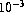but less than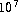, then it is represented as the integer part of m, in decimal form with no leading zeroes, followed by `'.'` (`\u002E`), followed by one or more decimal digits representing the fractional part of m.
• If m is less thanor not less than, then it is represented in so-called "computerized scientific notation." Let n be the unique integer such that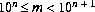; then let a be the mathematically exact quotient of m and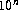so that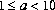. The magnitude is then represented as the integer part of a, as a single decimal digit, followed by `'.'` (`\u002E`), followed by decimal digits representing the fractional part of a, followed by the letter `'E'` (`\u0045`), followed by a representation of n as a decimal integer, as produced by the method `Integer.toString` of one argument (§20.7.12).
How many digits must be printed for the fractional part of m or a? There must be at least one digit to represent the fractional part, and beyond that as many, but only as many, more digits as are needed to uniquely distinguish the argument value from adjacent values of type `float`. That is, suppose that x is the exact mathematical value represented by the decimal representation produced by this method for a finite nonzero argument f. Then f must be the `float` value nearest to x; or, if two `float` values are equally close to x, then f must be one of them and the least significant bit of the significand of f must be `0`.

[This specification for the method `toString` is scheduled for introduction in Java version 1.1. In previous versions of Java, this method produces `Inf` instead of `Infinity` for infinite values. Also, it renders finite values in the same form as the `%g` format of the `printf` function in the C programming language, which can lose precision because it produces at most six digits after the decimal point.]

20.9.17 `public static Float valueOf(String s)throws NullPointerException, NumberFormatException`

The string `s` is interpreted as the representation of a floating-point value and a `Float` object representing that value is created and returned.

If `s` is `null`, then a `NullPointerException` is thrown.

Leading and trailing whitespace (§20.5.19) characters in `s` are ignored. The rest of `s` should constitute a FloatValue as described by the lexical syntax rules:

```FloatValue:
Signopt` `Digits` . `Digitsopt` `ExponentPartopt
Signopt `. `Digits` `ExponentPartopt
```
where Sign, Digits, and ExponentPart are as defined in §3.10.2. If it does not have the form of a FloatValue, then a `NumberFormatException` is thrown. Otherwise, it is regarded as representing an exact decimal value in the usual "computerized scientific notation"; this exact decimal value is then conceptually converted to an "infinitely precise" binary value that is then rounded to type `float` by the usual round-to-nearest rule of IEEE 754 floating-point arithmetic. Finally, a new object of class `Float` is created to represent this `float` value.

Note that neither `F` nor `f` is permitted to appear in `s` as a type indicator, as would be permitted in Java source code (§3.10.1).

20.9.18 `public boolean isNaN()`

The result is `true` if and only if the value represented by this `Float` object is NaN.

20.9.19 `public static boolean isNaN(float v)`

The result is `true` if and only if the value of the argument is NaN.

20.9.20 `public boolean isInfinite()`

The result is `true` if and only if the value represented by this `Float` object is positive infinity or negative infinity.

20.9.21 `public static boolean isInfinite(float v)`

The result is `true` if and only if the value of the argument is positive infinity or negative infinity.

20.9.22 `public static int floatToIntBits(float value)`

The result is a representation of the floating-point argument according to the IEEE 754 floating-point "single format" bit layout:

• Bit 31 (the bit that is selected by the mask `0x80000000`) represents the sign of the floating-point number.
• Bits 30-23 (the bits that are selected by the mask `0x7f800000`) represent the exponent.
• Bits 22-0 (the bits that are selected by the mask `0x007fffff`) represent the significand (sometimes called the mantissa) of the floating-point number.
• If the argument is positive infinity, the result will be `0x7f800000`.
• If the argument is negative infinity, the result will be `0xff800000`.
• If the argument is NaN, the result will be `0x7fc00000`.
In all cases, the result is an integer that, when given to the `intBitsToFloat` method (§20.9.23), will produce a floating-point value equal to the argument to `floatToIntBits`.

20.9.23 `public static float intBitsToFloat(int bits)`

The argument is considered to be a representation of a floating-point value according to the IEEE 754 floating-point "single format" bit layout. That floating-point value is returned as the result.

• If the argument is `0x7f800000`, the result will be positive infinity.
• If the argument is `0xff800000`, the result will be negative infinity.
• If the argument is any value in the range `0x7f800001` through `0x7fffffff` or in the range `0xff800001` through `0xffffffff`, the result will be NaN. (All IEEE 754 NaN values are, in effect, lumped together by the Java language into a single value called NaN.)
• In all other cases, let s, e, and m be three values that can be computed from the argument:
```
int s = ((bits >> 31) == 0) ? 1 : -1;
int e = ((bits >> 23) & 0xff);
int m = (e == 0) ?
(bits & 0x7fffff) << 1 :
(bits & 0x7fffff) | 0x800000;
```
Then the floating-point result equals the value of the mathematical expression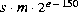.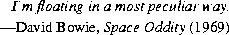Java Language Specification (HTML generated by Suzette Pelouch on February 24, 1998)
Copyright © 1996 Sun Microsystems, Inc. All rights reserved
Please send any comments or corrections to [email protected]